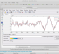## Matlab Loop Construction ?Started by 2 years ago5 replieslatest reply 7 months ago89 views

Hello,

Below is my current Matlab code. Wondering how to initially obtain analysis of the entire range and then only the new values. For example, I have a range of 4096 constantly  changing values. When a new value is introduced into the data set, an old value drops off. I am trying to loop through the entire data set one time initially and then only analyze and update the addition of the newest value to the data set. Currently, the code updates and radically changes the entire data set on every data update.

Thanks,

Richard

[xden,denoisedcfs] = wdenoise(MotorRPM, 6, 'No iseEstimate','LevelDependent', 'wavelet' ,'db9', 'denoisingmethod' ,'UniversalThreshold' ,'ThresholdRule' , 'Soft' ,);
tmpcfs = denoisedcfs;
for ii = [ 1 2 3 5 6 ]
tmpcfs{ii} = zeros(numel(tmpcfs{ii}),1);
end
decstruct = mdwtdec('c',MotorRPM,6,'db9');
decstruct.ca = tmpcfs{end};
decstruct.cd = tmpcfs(1:end-0);
W = mdwtrec(decstruct);
[ - ]Hi,

Actually as fas as I have understood from your code, you are trying wavelet denoising, hence your question is related to the applicability of wavelet analysis for the looping through the data as you have mentioned.

Wavelet analysis using the Matlab wavelet function you have used is done for the whole data. Do you need real time data analysis?

[ - ]Hello omersayli,

I did not get notice of your reply. Any way, yes, I am trying to obtain real time wavelet analysis. I don't want the whole data set to change each time the analysis is done. Trying to figure out how to run the analysis once on the whole data set and then only once on each new data value that comes in after that.Simple example below :

A

B       Run wavelet analysis once on A through D

C

D

E       Analyze new value "E" with wavelet and then add it to the data set A - D

Do this with each subsequent value after "E"....ie.."F", "G", "H", etc.

Thanks,

Richard

[ - ]Hi,

Actually I have always used Wavelet analysis for "off-line" analysis. Also the formulation of wavelet analysis somewhat requires whole data, think about the scaled versions of mother wavelet. You really need whole data.

One may think that -like short time fourier transform- you may do wavelet analysis for windows.  IN that case, windows should be large enough for proper wavelet analysis. But changing window one sample at a time is not useful I think.

[ - ]Hello omersayli,

You are indeed correct. I just tried one value and the analysis resulted in a rather small number that has no significance to my "pre -  analyzed" value. Thanks for your response / insight.

Richard

[ - ]